# High School Math : Solving Non-Quadratic Polynomials

## Example Questions

### Example Question #1 : Write A Polynomial Function From Its Zeros

Consider the equation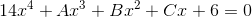.

According to the Rational Zeroes Theorem, if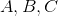are all integers, then, regardless of their values, which of the following cannot be a solution to the equation?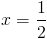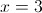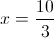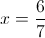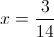Explanation:

By the Rational Zeroes Theorem, any rational solution must be a factor of the constant, 6, divided by the factor of the leading coefficient, 14.

Four of the answer choices have this characteristic: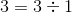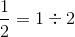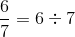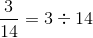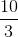is in lowest terms, and 3 is not a factor of 14. It is therefore the correct answer.